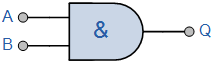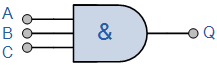A Logic AND Gate is a type of digital logic gate that has an output which is normally at logic level “0” and only goes “HIGH” to a logic level “1” when ALL of its inputs are at logic level “1”.

The output state of a “Logic AND Gate” only returns “LOW” again when ANY of its inputs are at a logic level “0”. In other words for a logic AND gate, any LOW input will give a LOW output.

The logic or Boolean expression given for a digital logic AND gate is that for Logical Multiplication which is denoted by a single dot or full stop symbol, ( . ) giving us the Boolean expression of:  A.B = Q.
Then we can define the operation of a 2-input logic AND gate as being:
“If both A and B are true, then Q is true”

2-input Transistor AND Gate

A simple 2-input logic AND gate can be constructed using RTL Resistor-transistor switches connected together as shown below with the inputs connected directly to the transistor bases. Both transistors must be saturated “ON” for an output at Q.Logic AND Gates are available using digital circuits to produce the desired logical function and is given a symbol whose shape represents the logical operation of the AND gate.

Digital Logic “AND” Gate Types

Symbol Truth Table2-input AND Gate B A Q 0 0 0 0 1 0 1 0 0 1 1 1 Boolean Expression Q = A.B Read as A AND B gives Q The 3-input Logic AND Gate Symbol Truth Table3-input AND Gate C B A Q 0 0 0 0 0 0 1 0 0 1 0 0 0 1 1 0 1 0 0 0 1 0 1 0 1 1 0 0 1 1 1 1 Boolean Expression Q = A.B.C Read as A AND B AND C gives Q

Because the Boolean expression for the logic AND function is defined as (.), which is a binary operation, AND gates can be cascaded together to form any number of individual inputs. However, commercial available AND gate IC’s are only available in standard 2, 3, or 4-input packages. If additional inputs are required, then standard AND gates will need to be cascaded together to obtain the required input value, for example.

Multi-input AND GateThe Boolean Expression for this 6-input AND gate will therefore be:   Q = (A.B).(C.D).(E.F)
If the number of inputs required is an odd number of inputs any “unused” inputs can be held HIGH by connecting them directly to the power supply using suitable “Pull-up” resistors.
Commonly available digital logic AND gate IC’s include:
TTL Logic AND Gates